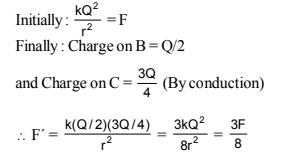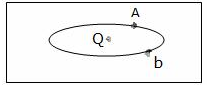Courses

# Test: Electrostatics Of Conductors

## 10 Questions MCQ Test Physics Class 12 | Test: Electrostatics Of Conductors

Description
This mock test of Test: Electrostatics Of Conductors for JEE helps you for every JEE entrance exam. This contains 10 Multiple Choice Questions for JEE Test: Electrostatics Of Conductors (mcq) to study with solutions a complete question bank. The solved questions answers in this Test: Electrostatics Of Conductors quiz give you a good mix of easy questions and tough questions. JEE students definitely take this Test: Electrostatics Of Conductors exercise for a better result in the exam. You can find other Test: Electrostatics Of Conductors extra questions, long questions & short questions for JEE on EduRev as well by searching above.
QUESTION: 1

### Two spherical conductors B and C having equal radii and carrying equal charges repel each other witha force F when kept apart at some distance. A third spherical conductor having same radius as that of B but uncharged is brought in contact with B, then brought in contact with C and finally removed awayfrom both. The new force of repulsion between B and C

Solution:QUESTION: 2

### As shown in the figure below, an ellipsoidal cavity is carved within a perfect conductor. A positive charge Q is placed at the centre of the cavity. If points A and b are shown on the cavity surface (see figure), then which among the following choices is correct?Solution:

Electric field at A is different from field at B because, E= K/r2
we know that conductor is an equipotential surface, so potential will be the same at A and B.
As charge density, σ∝1/r​, then charge density is different at A and B.

QUESTION: 3

### Electric field at the surface of a charged conductor is proportional to

Solution:

Electric field at the surfaces of charged conductors is σ/ε0​.n, where n is a unit vector normal to the surface.
We clearly see that the electric field is perpendicular to surface charge density (σ).

QUESTION: 4

Electrostatic field is zero_____ the conductor.

Solution:
QUESTION: 5

Inside a conductor, electrostatic field is:

Solution:

The electric field is zero inside a conductor Because the net charge inside a conductor remains zero , the total charge of a conductor resides on its surface , as charges want to attain equilibrium so they come on surface , to minimize the  repulsion among them .As the  charge inside a conductor is zero therefore , if we apply Gauss' theorem to find the electric field inside a conductor , we find it zero .

QUESTION: 6

Some charge is being given to a conductor. Then its potential:

Solution:

Given that some charge is given to a conductor then the whole charge is distributed over its surface only. Inside the conductor, the electric field is zero whereas potential is the same as on the surface. Hence, throughout the conductor, potential is the same i.e, the whole conductor is equipotential.

QUESTION: 7

The value of electric potential throughout the volume of a conductor is

Solution:

Since the electric field inside the conductor is zero and has no tangential component on its surface, therefore, no work is done in moving a test charge within the conductor or on its surface. It means the potential difference between any two points inside or on the surface is zero. Hence, electrostatic potential is constant throughout the volume of the charged conductor and has the same value on its surface as inside it.

QUESTION: 8

How does the charge densities of conductors vary on an irregularly shaped conductor?

Solution:

On an irregularly shaped conductor, the surface charge density is greatest at the locations where the radius of curvature of the surface is smallest i.e, charge density is inversely proportional to radius of curvature of surface. Since the radius of curvature is least at sharp portion and maximum at flat portion, so, charge density is high at sharp portions and less at flat portions.

QUESTION: 9

Two conductors having same type of charges are connected by a conducting wire. There would not be any amount of charges on them if:

Solution:

When there is potential difference across the conductor, the electric field is set up. Due to this charge will flow across the conductor. But when conductors have the same potential the charge will not flow from one conductor to the other.

QUESTION: 10

Consider a neutral conducting sphere. A positive point charge is placed outside the sphere. The net charge on the sphere is then,

Solution:

If a charge q is placed outside than the electric field lines incident on the conducting sphere , if some charge is developed due to thee lines than opposite surface becomes oppositely charge and the total charge becomes zero